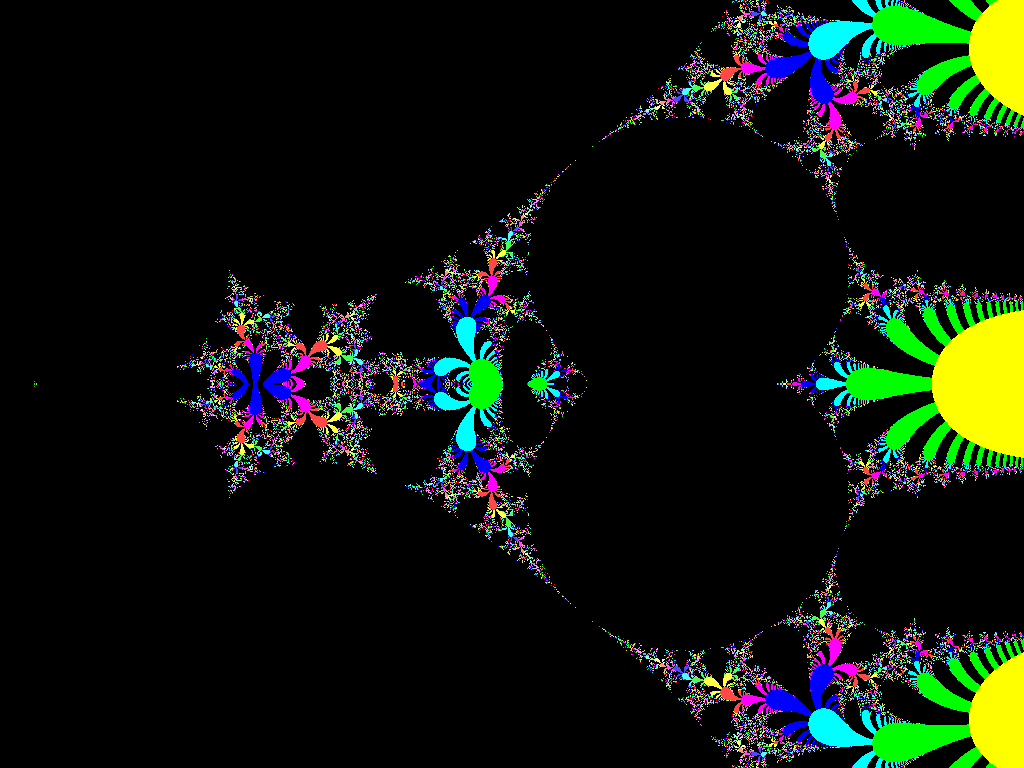# Some tetration you got there$\Large f(x;n) = \underbrace{ x^{x^{.^{.^x}}} }_{\text{number of } x \text{'s } =\ n }$


A positive integer $n$ is randomly chosen between 1 and 10000 inclusive for the function described above.

If $p$ denotes the probability that $\displaystyle \lim_{x \to 0} f(x;n) = 1,$ what is the value of $\left \lfloor 1000p \right \rfloor?$

×